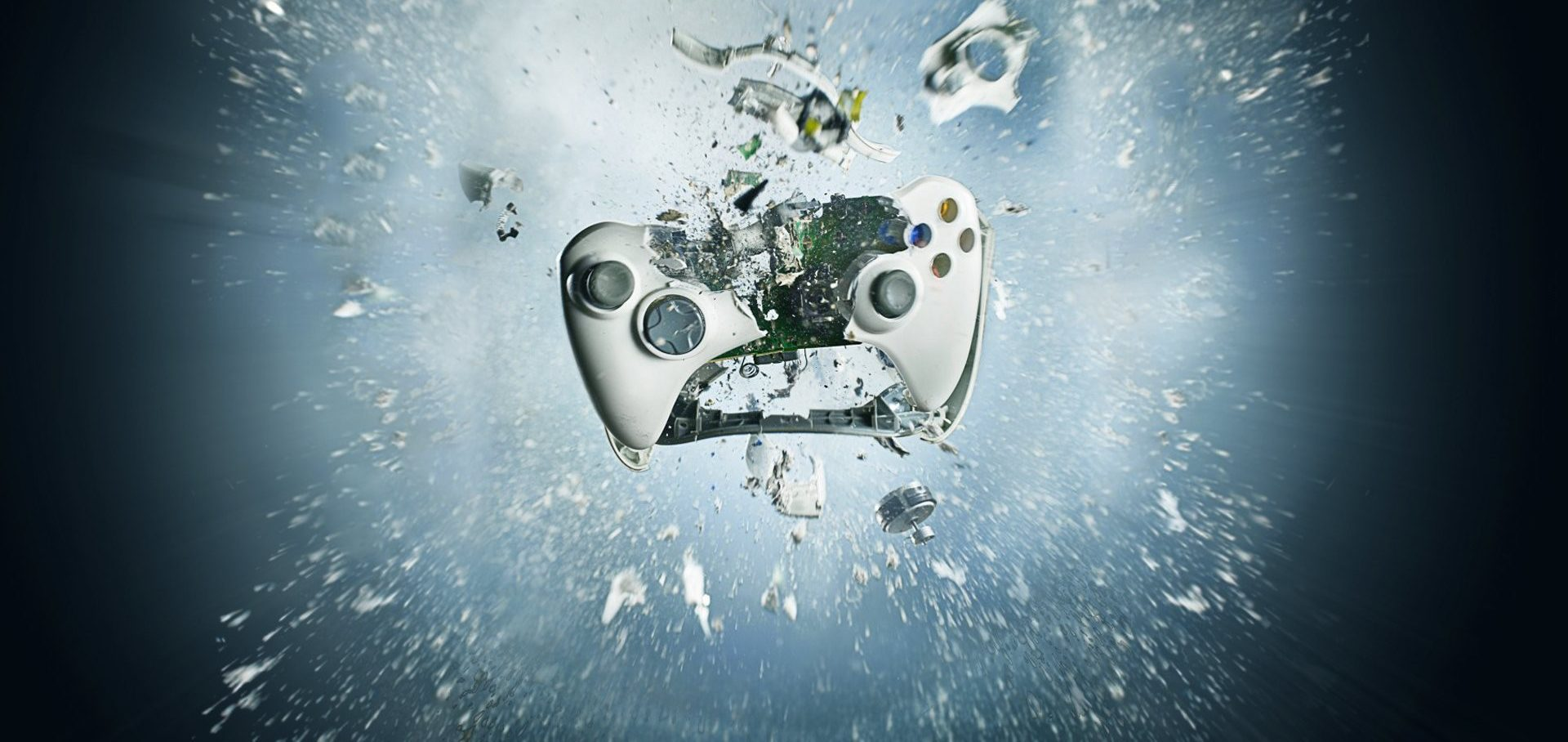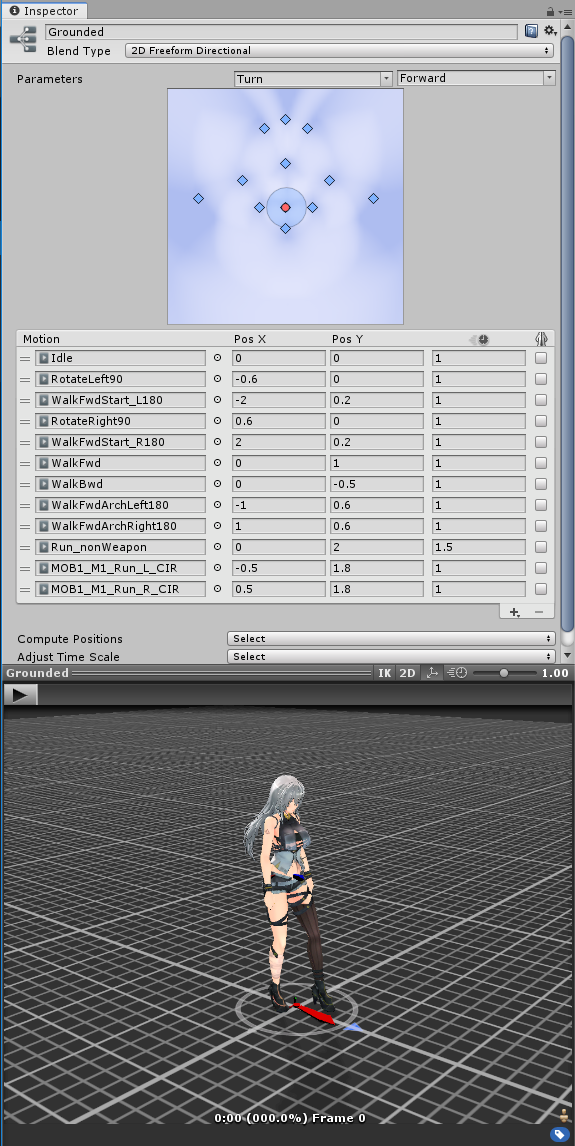# 我的人形控制開發筆記(一) Locomotion

## 使用環境假設

• 紅色的是鏡頭 Camera,
• 黃色的是我們的玩家代表物, , 上面紅格是正前向的方向代表.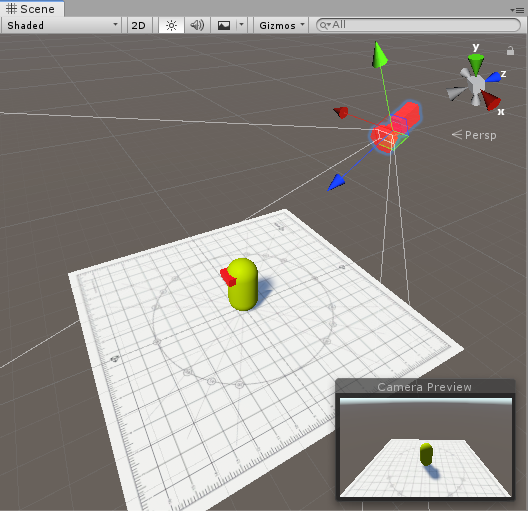• 在大部分時間 玩家代表物的方向 與 鏡頭方向不一致
• 頭會因應很多原因而改變, 也因此會影響玩家輸入的方式.
• 玩家普遍要求動作類遊戲的反應是一按下去立即進行.

## 所以 Locomotion 是甚麼?

• 靜止
• 步行
• 跑步

Unity3D 裡其實滿多範例解說的, 但其實 Unity 本身的範例有學習研究透徹的就會做出差不多這樣的圖.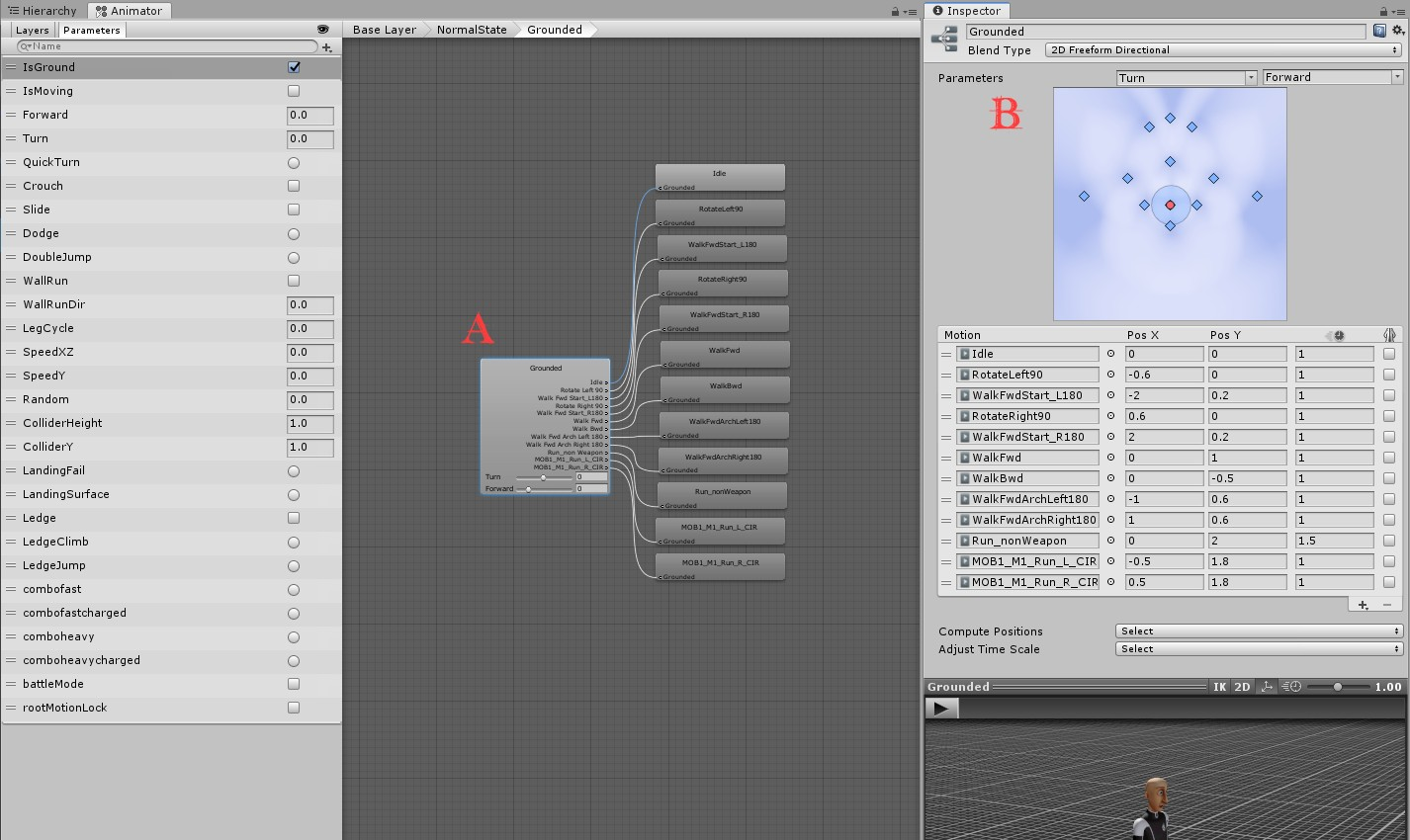## 方向?判斷玩家想去甚麼地方?

### 第一身視點的操作

• 控制扞 { 上, 下 } 代表人物的 { 前進, 後退 }
• 控制扞 { 左, 右 } 代表人物的 { 左移, 右移 }
```// convert 2D input into 3D direction, based on character current transform.
float horizontal = Input.GetAxis("Horizontal");
float vertical = Input.GetAxis("Vertical");
Vector3 direction = (transform.right * horizontal) + (transform.forward * vertical);
```

### 第三身視點的操作

e.g. 如下圖由右上角移到左下方.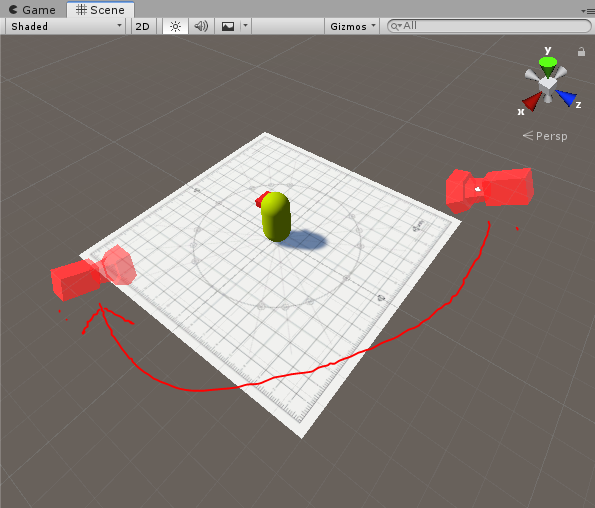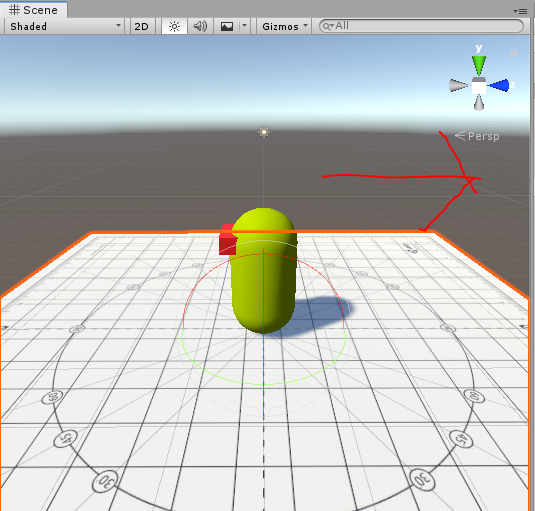1. 當玩家按下方向鍵 “右” 的時候, 從鏡頭的 “右” 方 (Local direction) 找到世界的指向 (World direction)
2. 再把這個 World direction, 以玩家的個人空間為準換算出是角式的 “後” 方.

```// based on avatar current standing plane, to calculate the cameraForward.
public Vector3 CameraForwardOnPlane => Vector3.ProjectOnPlane(Camera.main.transform.forward, transform.up).normalized;
public Vector3 CameraRightOnPlane => Vector3.ProjectOnPlane(Camera.main.transform.right, transform.up).normalized;```

``` // Convert 2D input into 3D local direction
float Horizontal = Mathf.Clamp(Input.GetAxis("Horizontal"), -1f, 1f);
float Vertical = Mathf.Clamp(Input.GetAxis("Vertical"), -1f, 1f);
Vector3 worldDirection = (Horizontal * CameraRightOnPlane) + (Vertical * CameraForwardOnPlane);```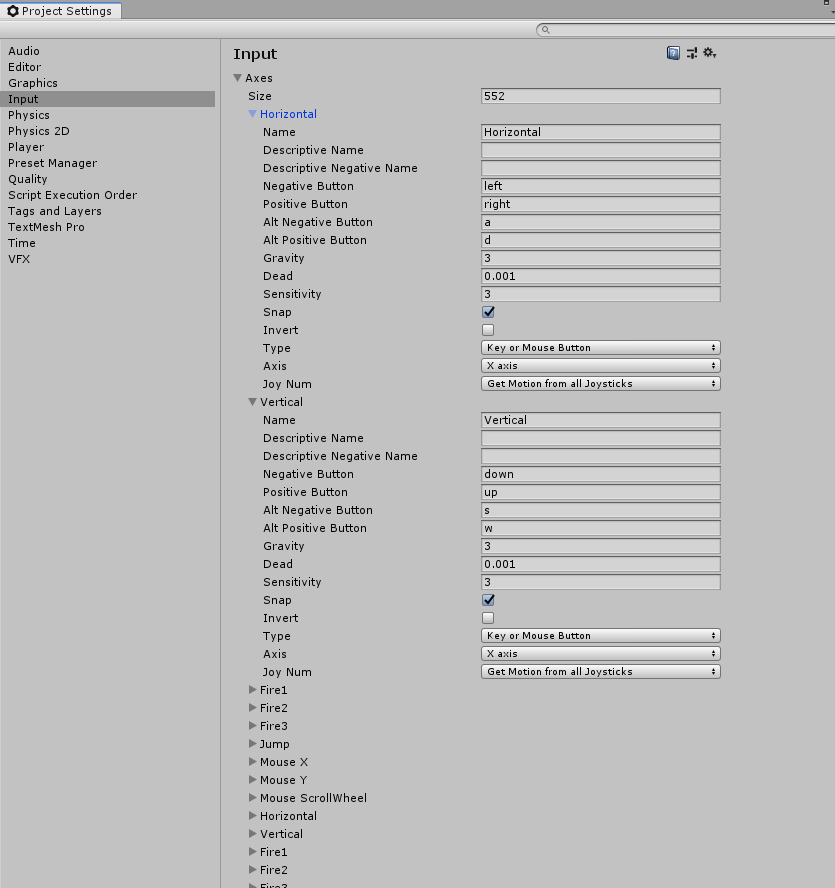```float maxSpeed = 3f;
transform.position += WorldDirection * (maxSpeed * Time.deltaTime); // 現在的地點, 向世界方向移動這個距離.```

“WorldDirection” 是一支長度 <= 1f 的矢量,用以代表使用者的輸入強度.

## 移動可以了,但動作怎樣處理?

```public Transform playerTransform; // assume it's assigned in inspector!
/////////
void Update()
{
Vector3 localDir = playerTransform.InverseTransformVector(worldDirection); // world -> local
animator.SetFloat("MoveX", localDir.x);
animator.SetFloat("MoveY", localDir.z); // Z not Y
}```

e.g. 自己的前方, 跟 世界北方 之間的關係.

```public float maxSpeed = 3f;

// based on avatar current standing plane, to calculate the cameraForward.
// Caution : Camera.main are costly API call(GameObject.Find + tags matching), consider cache result per frame.
public Vector3 CameraForwardOnPlane => Vector3.ProjectOnPlane(Camera.main.transform.forward, transform.up).normalized;
public Vector3 CameraRightOnPlane => Vector3.ProjectOnPlane(Camera.main.transform.right, transform.up).normalized;

private void Update()
{
// Convert 2D input into 3D local direction
float Horizontal = Input.GetAxis("Horizontal");
float Vertical = Input.GetAxis("Vertical");
Vector3 worldDirection = (Horizontal * CameraRightOnPlane) + (Vertical * CameraForwardOnPlane);

// Optional : to prevent out of range on some device
worldDirection = Vector3.ClampMagnitude(worldDirection, 1f);

// Move
// if (worldDirection.sqrMagnitude > 0.01f) // Option B, when length of vector are represent the movement distance
if (worldDirection != Vector3.zero)
{
transform.position += worldDirection * (maxSpeed * Time.deltaTime);
transform.forward = worldDirection;
}

// An update animator parameters
Vector3 localDir = transform.InverseTransformVector(worldDirection); // world -> local
animator.SetFloat("MoveX", localDir.x);
animator.SetFloat("MoveY", localDir.z); // Z not Y
}```